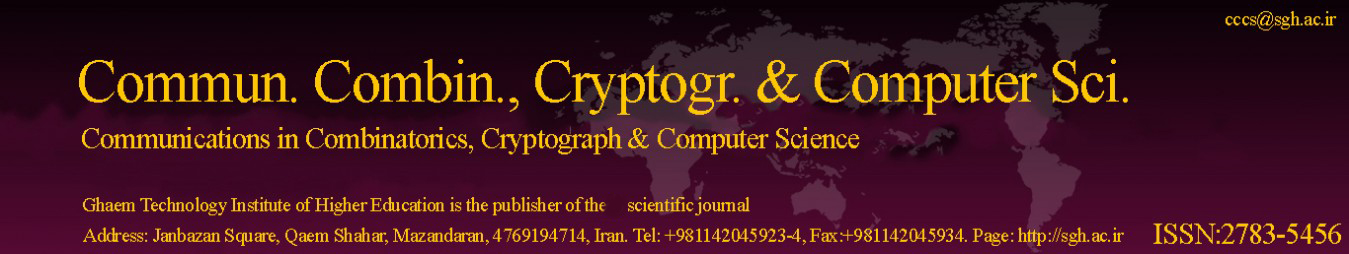Original Research Article

Article volume = 2021 and issue = 2

Pages: 179–185

Article publication Date: September 17, 2022

# First KCD Matrix and its Laplacian Energy

#### Keerthi G. Mirajkar and Akshata Morajkar

Department of Mathematics, Karnatak University’s Karnatak Arts College, Dharwad - 580001, Karnataka, India.

##### Abstract:

This article introduces the concept associated with Laplacian energy for first KCD matrix named as first KCD Laplacian energy. Further, we investigate some basic results related to this concept and also develop significant bounds for first KCD Laplacian energy.

##### Keywords:

First KCD Laplacian matrix, first KCD Laplacian eigenvalues, first KCD Laplacian energy.

##### References:
•  R. Balakrishnan, The energy of a graph, Linear Algebra Appl., 387 (2004), 287–295. 1
•  S. Bernard, J. M. Child, Higher Algebra, Macmillan India Ltd., New Delhi, (2001). 1
•  I. Gutman, The energy of a graph, Ber. Math. Stat. Sekt. Forschungsz. Graz, 103 (1978), 1–22. 1
•  I. Gutman, B. Zhou, Laplacian energy of a graph, Linear Algebra Appl., 414 (2006), 29–37. 1
•  F. Harary, Graph Theory, Addison – Wesely, Reading, Mass, (1969). 1
•  G. Indulal, I. Gutman, A. Vijaykumar, On distance energy of graphs, MATCH Commun. Math. Comput. Chem., 60 (2008), 461–472. 1
•  K. G. Mirajkar, A. Morajkar, First KCD matrix and first KCD energy of a graph, Accepted for publication in Mathematical Forum, 28 (2020). 1
•  B. Zhou, I. Gutman, T. Aleksic, A note on Laplacian energy of graphs, MATCH Commun. Math. Comput. Chem., 60 (2008), 441–446. 1
•  B. Zhou, More on energy and Laplacian energy, MATCH Commun. Math. Comput. Chem., 64 (2010), 75–84. 1